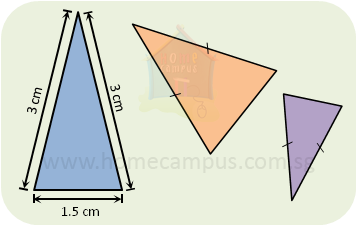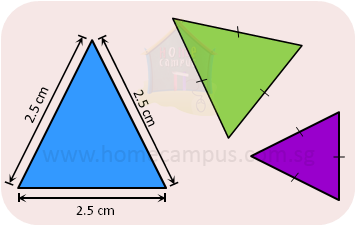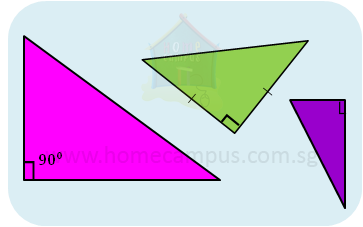## What are Different Types of Triangles?

Practice Unlimited Questions

#### TRIANGLES

This lesson covers the following types of triangles:

#### 1. Isosceles Triangles

A triangle that has two equal sides is an isosceles triangle.Each triangle above has two equal sides.
Hence, they are all isosceles triangles.
We show two or more equal sides by putting a small slash across them.

Trivia:

If you measure the angles of an isosceles triangle you will notice that the angles opposite its equal sides are equal.

#### 2. Equilateral Triangles

A triangle that has all equal sides is an equilateral triangle.Each triangle above has all 3 sides of the same length.
Therefore, they are all equilateral triangles.
We show an equilateral triangle by putting a small slash across each of its 3 sides.

Trivia:

If you measure the angles of an equilateral triangle you will notice that each of its angles is equal to 60°.

#### 3. Right-angled Triangles

A right-angled triangle, as the name suggests, is a triangle that has one angle that is equal to 90°.Each triangle above has one angle that is a right angle, that is, 90°.
So, they are all right-angled triangles.

Trivia:

If you measure the other two angles of a right-angled triangle you will notice that their sum is equal to 90°.